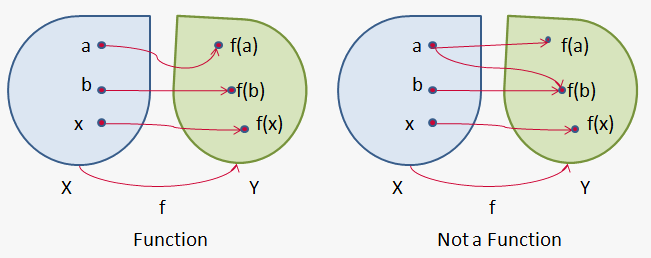# Definition of Function

Suppose we are given two variables  x  and y with domain of variation X and Y respectively.

Suppose that x can take any value from X without any restrictions. Then variable y is called function of variable x in domain of its variation X, if according to some law or rule we can assign to each value of x from X one definite value of y from Y.

Independent variable x is also called argument of function.

Set X of values that can take independent variable x is called domain of function.

Note that sequence is particular case of function where domain is set of all natural numbers.

Set Y of values that can take dependent variable y is called range of function.

When we define a function we don't explictly state range of function, because it is automatically defined from domain of function and law of correspondence between x and y.

In definition we wrote that we assign to each value of x one value of y. Actually we can to each value of x assign more than one value of y. Such function is called multi-valued. In calculus we will consider only one-valued functions and even don't treat multi-valued function as function (see figure: to the right is not a function because when x=a y takes two values).To write that y is a function of x we write that y=f(x),y=phi(x),y=F(x) etc.

Letters f,phi,F,.. characterize rule that allows for every value of x find corresponding value of y. Therefore, if we consider different functions of same argument x then we should use different letters to denote them.

Of course we can use any letter to write functional dependence. Often we can even repeat letter y: y=y(x).

If we consider function y=f(x) and want to write its particular value when x takes particular value x_0, we write f(x_0).

For example, if f(x)=1/(1+x^2), g(t)=10/t, h(u)=sqrt(1-u^2) then

when x=1 f(1)=1/(1+1^2)=1/2; when t=5 g(5)=10/5=2; when u=a+b h(a+b)=sqrt(1-(a+b)^2).